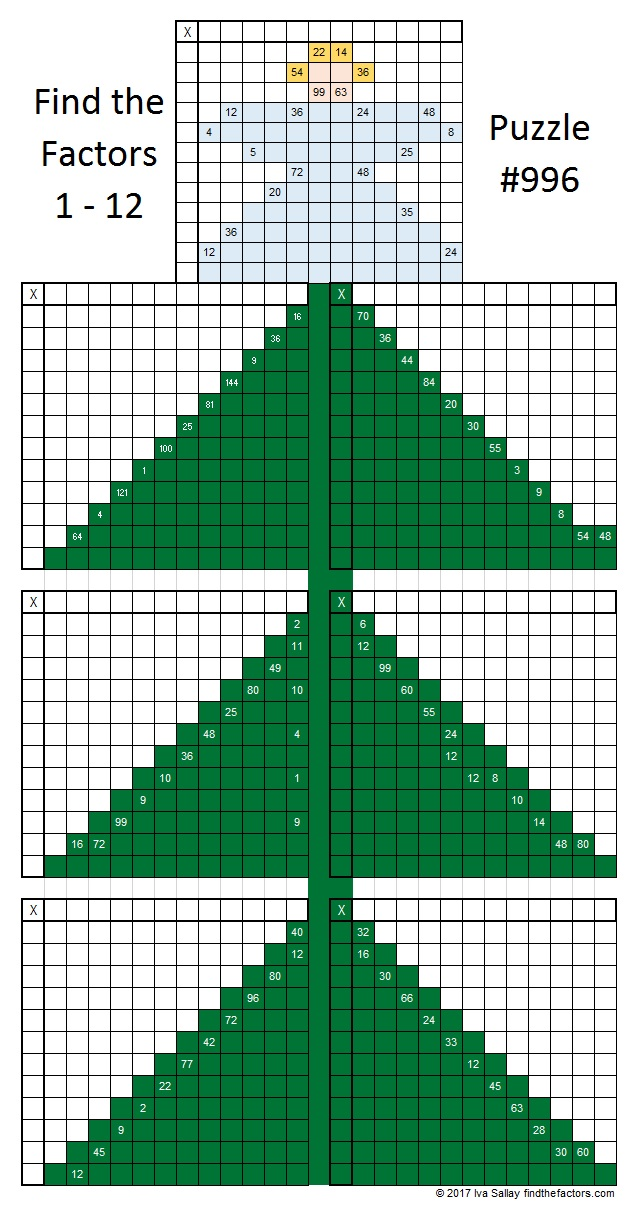# 996 Christmas Factor Tree

The puzzles this week might look rather plain, but together the seven puzzles make a lovely Christmas tree factoring puzzle. The difficulty level of each of the puzzles is not identified. Some of them are very easy, and some of them are difficult. Some are in-between. How many of them can you solve?Print the puzzles or type the solution in this excel file: 12 factors 993-1001

Here’s a little about the number 996:

Here are a few of its possible factor trees. They look a little like Christmas trees, too.Usually,  I only go up to base 36 when I look for palindromes or repdigits. 966 is NOT a palindrome or repdigit in any of those bases, but can it ever be one? To me, repdigits are more interesting than palindromes because you can find them by factoring.  966 has 6 factors greater than 36: 83, 166, 249, 332, 498, 996. If you subtract 1 from each of those, then 996 will be a repdigit in each of those bases.
In BASE 82, it’s CC (C is 12 base 10)
In BASE 165, it’s 66
In BASE 248, it’s 44
In BASE 331, it’s 33
In BASE 497, it’s 22
In BASE 995, it’s 11
Don’t be surprised when I tell you that 12, 6, 4, 3, 2, and 1 are also factors of 996!

• 996 is a composite number.
• Prime factorization: 996 = 2 × 2 × 3 × 83, which can be written 996 = 2² × 3 × 83
• The exponents in the prime factorization are 2, 1, and 1. Adding one to each and multiplying we get (2 + 1)(1 + 1)(1 + 1) = 3 × 2 × 2 = 12. Therefore 996 has exactly 12 factors.
• Factors of 996: 1, 2, 3, 4, 6, 12, 83, 166, 249, 332, 498, 996
• Factor pairs: 996 = 1 × 996, 2 × 498, 3 × 332, 4 × 249, 6 × 166, or 12 × 83,
• Taking the factor pair with the largest square number factor, we get √996 = (√4)(√249) = 2√249 ≈ 31.55947This site uses Akismet to reduce spam. Learn how your comment data is processed.